# alysoid

## exponentionalwith - π/2a < t < π/2a

The alysoid 1) is a generalization of the catenary (a=1).
However, sometimes the alysoid is used as an alternative name for this catenary.

For a=2 the curve can be used to find an optimal acceleration profile.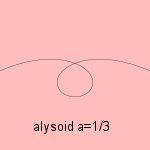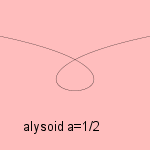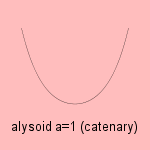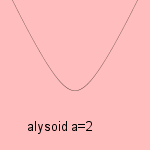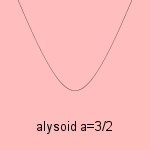The alysoid can be defined as the curve for which the center of curvature describes the path of a parabola, when rolling over a straight line (with the parabola perpendicular to the straight line).

It was Césaro who started to study the curves, in 1886.

The intrinsic Whewell equation of the alysoid has a rather simple form: s = tan aφ, s being the arc length.

notes

1) Alusion (Gr.) = little chain.